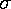## Student's t-Tests

"Student" (real name: W. S. Gossett [1876-1937]) developed statistical methods to solve problems stemming from his employment in a brewery. Student's t-test deals with the problems associated with inference based on "small" samples: the calculated mean (Xavg) and standard deviation () may by chance deviate from the "real" mean and standard deviation (i.e., what you'd measure if you had many more data items: a "large" sample). For example, it it likely that the true mean size of maple leaves is "close" to the mean calculated from a sample of N randomly collected leaves. If N=5, 95% of the time the actual mean would be in the range: Xavg± 2.776/N1/2 ; if N=10: Xavg± 2.262/N1/2 ; if N=20: Xavg± 2.093/N1/2 ; if N=40; Xavg± 2.023/N1/2 ; and for "large" N: Xavg± 1.960/N1/2 . (These "small-sample" corrections are included in the descriptive statics report of the 95% confidence interval.)

### Are two sets of data really different?

If we have two collections of maple leaves (i.e., two samples), it is quite likely that in detail the collections are different: different highs, lows, and average leaf sizes. Is the measured difference in average leaf size large enough that we should reject the null hypothesis that in fact such differences are due to "chance"? Given the above sort of information on the likely range for the actual mean of each sample, the question basically reduces to whether the likely ranges overlap (in which case the means could be the same: in the overlap of the intervals, and we may not reject the null hypothesis) or if they do not overlap (in which case we must reject the null hypothesis: the difference is most likely not due to chance). To report the variety of possible outcomes: from means not "significantly" different to means in fact "significantly" different, the probability that the difference is due to chance is reported. Reject the null hypothesis if P is "small".

### Paired Data

Very often the two samples to be compared are not randomly selected: the second sample is the same as the first after some treatment has been applied.

Cedar-apple rust is a (non-fatal) disease that affects apple trees. Its most obvious symptom is rust-colored spots on apple leaves. Red cedar trees are the immediate source of the fungus that infects the apple trees. If you could remove all red cedar trees within a few miles of the orchard, you should eliminate the problem. In the first year of this experiment the number of affected leaves on 8 trees was counted; the following winter all red cedar trees within 100 yards of the orchard were removed and the following year the same trees were examined for affected leaves. The results are recorded below:

```tree    number of rusted     number of rusted    difference: 1-2
leaves: year 1       leaves: year 2

1            38                   32                6
2            10                   16               -6
3            84                   57               27
4            36                   28                8
5            50                   55               -5
6            35                   12               23
7            73                   61               12
8            48                   29               19

average        46.8                 36.2             10.5
standard dev   23                   19               12```
As you can see there is substantial natural variation in the number of affected leaves; in fact, a unpaired t-test comparing the results in year 1 and year 2 would find no significant difference. (Note that an unpaired t-test should not be applied to this data because the second sample was not in fact randomly selected.) However, if we focus on the difference we find that the average difference is significantly different from zero. The paired t-test focuses on the difference between the paired data and reports the probability that the actual mean difference is consistent with zero. This comparison is aided by the reduction in variance achieved by taking the differences.